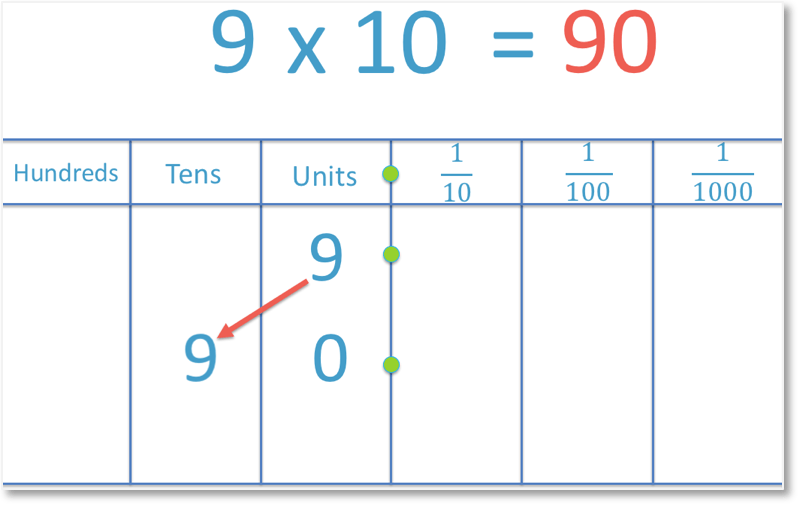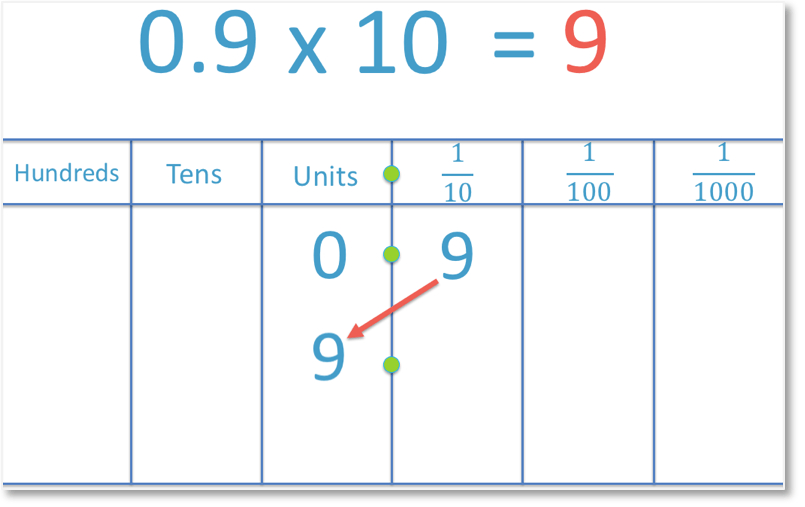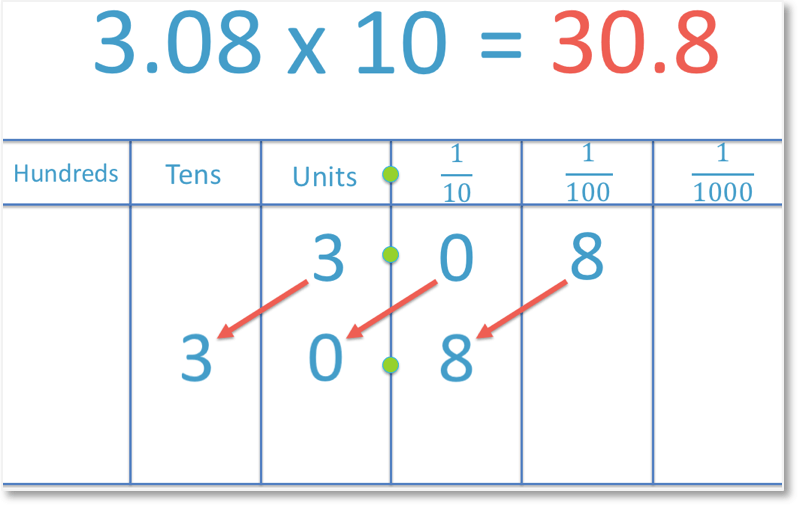# Multiplying by 10

Multiplying by 10• When multiplying a number by 10 we move each digit in the number one place value column to the left.
• 9 contains the digit of ‘9’ in the units (or ones) column.
• When we multiply 9 by 10 we move the digits one place value column to the left.
• The ‘9’ digit moves from the units column into the tens column.
• We put a ‘0’ in the units column since we have moved the ‘9’ digit out of this column.
• 9 x 10 = 90.
• If we are multiplying a whole number by 10, then we add the digit ‘0’ to the end of our number.
To multiply a number by 10 move each digit one place value column to the right.

To multiply a whole number by 10 then add the digit ‘zero’ to the end of the number.• We will multiply the decimal 3.08 by 10 by considering place value columns.
• To multiply a number by ten, move all digits one place value column to the left.
• The ‘3’ digit moves from the units column to the tens column.
• The ‘0’ digit moves from the tenths column to the units column.
• The ‘8’ digit moves from the hundredths column to the tenths column.
• 3.08 x 10 = 30.8 and we can see the the digits of ‘3’, ‘0’ and ‘8’ remain in this order.Supporting Lessons#### Multiplying by Ten: Interactive Questions

Multiplying Decimals by 10: Interactive Questions

# How do we Multiply a Number by Ten?

To multiply a number by 10 move each digit in that number one place to the left.

If there is no longer a digit in any of the columns to the left of the decimal point, put a zero in these columns. These are the units columns, tens columns, hundreds columns and so on.

To multiply a whole number by ten, add a zero to the end of the number.

This is a commonly taught technique for multiplying by ten, however this will only work for whole numbers and will not work for decimals.

For example, we will multiply the whole number 9 by ten.In the example above, the 9 has been moved one place value column to the left. The digit of ‘9 is moved from the units column into the tens column.

Because there is now a space in the units column, a zero is written in to show that the units column contains nothing.

Since 9 is a whole number, it is much easier to put a zero on the end of the digit ‘9’ to make ’90’.

We might also know from our times tables that 9 x 10 = 90.

However, it is important to understand how multiplying by 10 works because not all numbers that we will be multiplying will be whole numbers and we will use this same method for decimal numbers.

Here is an example of multiplying a decimal by 10:To multiply the decimal 0.9 by 10, we move the digit of 9 one place to the left. So we move the nine from the tenths column to the units column. Therefore, 0.9 x 10 = 9.

We do not write any zeros after a decimal point unless there are other non-zero digits that come after the decimal point.

In the following example, we are asked to multiply the decimal number 0.009 by 10.We begin by moving the digit of 9 one place to the left, from the thousandths column to the hundredths column. We then make sure that we show that the units column and the tenths column are both worth zero by writing a zero in each space.

We always put zeros in between the decimal point and our first digit that is not zero.

We also always write the zero before the decimal point if there are no digits to the left of the decimal point at all.

For instance we wrote 0.09 rather than .09.

When multiplying a number with more than one digit by 10, we need to make sure that we move every digit one place value column to the left and that the digits remain in the exact same order.

For example:To multiply the decimal number of 4.1 by 10, we begin by moving the 4 one place to the left. So we move it from the units column to the tens column.

The digit of 1 can then follow. We move the 1 from the tenths column to the units column. And so, 4.1 x 10 = 41.

Notice how the two digits in 4.1 were ‘4’ and ‘1’ in that order and they remain next to each other in the answer of 41.

Since the answer of 41 is a whole number, we do not need to write a zero after the decimal point.

We need to be more careful when multiplying by 10 where there is a zero between two other digits.

For example:We must make sure that we move every digitin the decimal 3.08 one place to the left, including the zero. We move the 3 from the units column to the tens column, we move the 0 from the tenths column to the units column and we move the 8 from the hundredths column to the tenths column.

The digits remain in the same order. Therefore, 3.08 x 10 = 30.8.

A trick for teaching multiplying by ten is that instead of moving the digits one place to the left, we move the decimal point one place to the right.

Technically, this is not what is happening, however this can be used as a trick to help students who find the original process more challenging. This is because it can be easier to move one decimal point right rather than moving every digit to the left in a longer number.

When multiplying a decimal number by ten mentally, moving the decimal point may be a suitable process to use, however it is worth remembering that it is actually the digits that are moving.Now try our lesson on Multiplying by 100 where we learn how to multiply a whole number and a decimal number by 100.